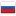Центроидная декомпозиция на графе с циклами

Revision en1, by dimss, 2023-01-26 15:34:26

It seemed to me that this was nowhere to be found and I came up with something new, but after I proposed a task on codeforces, one of the coordinators said that a couple of years ago he had already seen a task where something similar was used.

## Problem

Let some problem be given that we can solve using centroid decomposition. For example, like this. Given a tree, for each vertex assigned number a[i]. There are 2 types of queries.

1. Given $v$, $d$, $c$. You are to recolor all vertices at a distance no greater than $d$ from vertex v in color $c$.

2. Given $v$. We need to find out the color of vertex $v$.

Now this task is not on a tree, but on a connected graph. But there is one very interesting limitation: m — n + 1 $\le$ 100. hat is, no more than 100 edges were added to the tree.

First, let me remind you how the problem was solved on the tree. We built a tree of centroids of depth $O(\log n)$, for each centroid we stored a structure that could color vertices from $C$ at a distance of no more than $d$ and recognize the color of the vertex. This structure is called a stack, which stores triplets of elements (distance, color, time). Need to mention, that the distance strictly decreases. Then, when painting, you need to remove some elements from the end of the stack and put a new element. So, when requesting a color, the answer can be found by binary search by local depth $v$.

We have learned to respond to requests if we paint vertices from a given centroid. Now let's go through all the centroids $C$, which are the ancestors of $v$ in the centroid tree, and for them we will perform the operations above. If the query is of the first type, and we paint from $v$ at a distance of no more than $d$, then from $C$ we need to paint at a distance of at most $d−dist(v,C)$ If we are now answering a query of the second type, then from all the colors we need to choose the one which has the maximum time. Why does it work? Suppose there was a paint request from $v$ that hit $u$, whose color we now want to know. Then there is a centroid $C=lca(v,u)$ in the tree of centroids. $C$ is a common centroid for both $v$ and $u$. Moreover, it is on the path from $v$ to $u$ in the source tree. Then when we iterate over $C$, we are guaranteed the correct answer.

Now, when we have not a tree, but a graph, it is impossible to build a tree of centroids. Let's rewrite the definition of centroid and build something similar. get(G) will produce a new centroid according to the following algorithm:

1. If G has a cycle, then return any vertex on the cycle.

2. If G is a tree, then return any ordinary centroid.

Another change in the construction is that now the depths of the vertices must be searched not with dfs, but with bfs. Then the tree of new centroids will look like a bamboo of 100 vertices, from which the tree of ordinary centroids is suspended. I claim that then the algorithm above will work correctly. Again, suppose there was a coloring in $v$ that hits $u$, and we want to know the color of $u$. Then there is a vertex $C$ that is an ancestor of $lca(v,u)$ (note that not exactly lca, but an ancestor of lca) such that the shortest path from $v$ to $u$ passes through vertex $C$ and when , the correct answer will be calculated. And when we sort it out, the correct answer will be calculated.

Processing the queries of first type amortized takes $O(m−n+1+log n)$, and the second $O((m−n+1+log n)log n)$.

## Other problems

All the tasks that I know and know how to solve, where centroids are used to answer queries (there are not very many of them), I know how to generalize to a graph. But, unfortunately, not every task on the tree can be generalized in this way. For example, if centroids are used to count the number of paths in the tree (more precisely, the number of pairs of $v$, $u$), then some paths will be counted several times if there are several extreme paths between them. The answer obtained in this way gives a good upper bound. The real answer is less than no more than $(m - n + 1)n$.

#### History

RevisionsRev. Lang. By When Δ Comment
en6dimss 2023-01-26 15:41:17 4
en5dimss 2023-01-26 15:40:16 78
en4dimss 2023-01-26 15:39:27 0 (published)
ru5dimss 2023-01-26 15:38:12 0 (опубликовано)
en3dimss 2023-01-26 15:37:35 73
ru4dimss 2023-01-26 15:37:00 73
en2dimss 2023-01-26 15:35:34 140
en1dimss 2023-01-26 15:34:26 4147 Initial revision for English translation (saved to drafts)
ru3dimss 2023-01-26 12:03:12 5 Мелкая правка: 'дел задачу использов' -> 'дел задачу, где использов'
ru2dimss 2023-01-26 11:57:44 3840 Мелкая правка: 'm - n + 1 ≤≤\leq 100. То е' -> 'm - n + 1 $\le$ 100. То е'
ru1dimss 2023-01-26 10:52:14 54 Первая редакция (сохранено в черновиках)